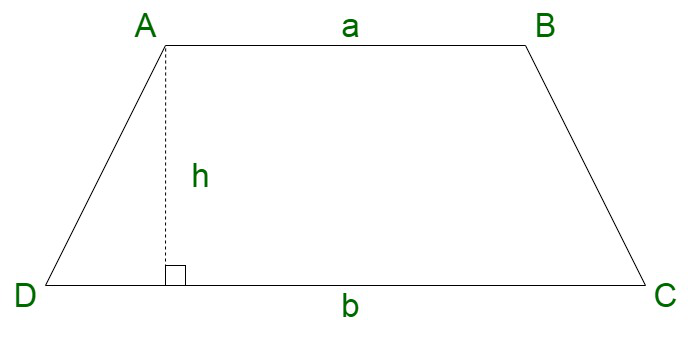GeeksforGeeks App
Open AppBrowser
Continue

# How to find the perimeter of a trapezium?

Trapezium is a geometrical figure containing one pair of opposite sides to be parallel in nature. These parallel sets of sides are called bases and the non-parallel sides of a trapezium are called legs. It can be considered as a special case of a parallelogram with two parallel sides. Also referred using the name trapezoid.

Types of Trapezium

The trapezium can be categorised into the following three types:

• Isosceles Trapezium
• Scalene Trapezium
• Right Trapezium

Properties of Trapezium

A trapezium is associated with the following attributes :

• One pair of opposite sides are parallel.
• Unequal non-parallel sides. Exception is the isosceles trapezium.
• The diagonals intersect each other.
• The line that intersects the mid-points of the non-parallel sides is always parallel to the bases. These are equal to half of the sum of parallel sides

Perimeter of Trapezium

The total summation of the length of the boundary formed by the sides of the trapezoid or trapezium is referred to as the perimeter of the trapezium. It is an irregular polygon. The perimeter of the trapezoid is contained in the two-dimensional shape since all the lines and vertices are contained in the two-dimensional plane only. The unit of the perimeter of the trapezoid is referred by the following units, such as “inches”, “feet”, “metres” etc.

Perimeter of trapezium = Sum of non-parallel sides + Sum of parallel sides

### How to Find the Perimeter of Trapezoid?

The perimeter of the trapezoid can be found by performing the following steps:

Step 1: Compute the dimensions of all the sides of the trapezoid.

Step 2: Perform the summation of the lengths of all the sides.

Step 3: Compute its units.

### Formula of Perimeter of Trapezoid

Let us assume a trapezoid ABCD, where we have sides AB and CD parallel to each other. They form the pair ‘bases’ while AD and BC are non-parallel sides (that is, they are legs). Let us assume the perimeter of the trapezoid to be equivalent to “P”. units.The perimeter of trapezoid ABCD is given by,

P = AB + BC + CD + AD.

The perimeter of the trapezoid in terms of area is given by the following formula,

P = (sum of the lengths of non-parallel sides) + 2where A is the area of trapezoid and h is its height.

### Sample Questions

Question 1. Calculate the perimeter of a trapezium having parallel sides 4 cm and 6 cm. The non-parallel sides 5 cm and 8 cm?

Solution:

Here we have,

The parallel sides are 4 cm and 6 cm

The non-parallel sides are 5 cm and 8 cm

To find the perimeter of the trapezium we need to add all the sides of the trapezium.

Perimeter of trapezium = Sum of parallel sides + sum of non-parallel sides

Perimeter of trapezium = 4 cm + 6 cm + 5 cm + 8 cm

Perimeter of trapezium = 23 cm

Therefore,

Perimeter of trapezium is 23 cm.

Question 2. Calculate the perimeter of a trapezium ground having parallel side 20 m 25 m and non-parallel side 18 m and 16 m?

Solution:

Here we have to find the perimeter of the trapezium ground

We have,

The parallel sides are 20 m and 25 m

The non-parallel sides are 18 m and 16 m

To find the perimeter of the trapezium ground we need to add all the sides of the trapezium ground.

Perimeter of trapezium ground = Sum of parallel sides + sum of non-parallel sides

Perimeter of trapezium ground = 20 m + 25 m + 18 m + 16 m

Perimeter of trapezium ground = 79 m

Therefore,

Perimeter of trapezium ground is 79 cm.

Question 3. If the perimeter of the trapezium is 54 cm and the parallel sides are 10 cm and 15 cm. Assume that the non-parallel sides are equal. Then find the length of both of the non-parallel sides?

Solution:

Here we have the perimeter of the trapezium 54 cm

We are given that the parallel sides are 10 cm and 15 cm

The non-parallel sides are equal

Assume that the non-parallel side is x.

Therefore,

The perimeter of the trapezium = 54 cm

The perimeter of the trapezium = 10 cm + 15 cm + x + x

54 = 25 + 2x

2x = 54 – 25

2x = 29

x =x = 14.5 cm

Therefore the non-parallel side of the trapezium is 14.5 cm

As both the sides are equal

Hence,

Sides of the trapezium = 10 cm, 15 cm, 14.5 cm, 14.5 cm

Question 4. Calculate the perimeter of the regular trapezium having the parallel side 15 cm 17 cm, the length of the non-parallel side is 8 cm.

Solution:

Here we are given,

Parallel side of the trapezium are 15 cm and 17 cm

Length of the non-parallel side is 8 cm, 8 cm

Therefore,

Perimeter of the trapezium = Sum of all the sides of the trapezium

Perimeter of the trapezium = 15 cm + 17 cm + 2(8)

= 15 cm + 17 cm + 16 cm

Perimeter of the trapezium = 48 cm

Question 5. Calculate the perimeter of a trapezium having parallel sides 25 cm and 30 cm. The non-parallel sides 19 cm and 18 cm?

Solution:

Here we have,

The parallel sides are 25 cm and 30 cm

The non-parallel sides are 19 cm and 18 cm

To find the perimeter of the trapezium we need to add all the sides of the trapezium.

Perimeter of trapezium = Sum of parallel sides + sum of non-parallel sides

Perimeter of trapezium = 25 cm + 30 cm + 19 cm + 18 cm

Perimeter of trapezium = 92 cm

Therefore,

Perimeter of trapezium is 92 cm.

My Personal Notes arrow_drop_up
Related Tutorials